# Zassenhaus conjecture

(diff) ← Older revision | Latest revision (diff) | Newer revision → (diff)

Just as the only roots of unity in a cyclotomic field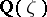are of the form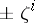, there is the classical theorem of G. Higman stating that the torsion units in the integral group ring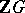of a finite Abelian group are of the form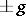,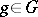. Of course, ifis non-Abelian, then any conjugate ofis also of finite order; however, these are not all the torsion units in. The famous Zassenhaus conjecture says that for a finite groupall torsion units ofare rationally conjugate to,:

ZC1) Let,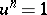for some; then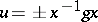for someand some unit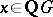. This conjecture was proved to be true by A. Weiss, first for-groups [a16] and then for nilpotent groups [a17] (cf. also Nilpotent group). In fact, Weiss proved the following stronger Zassenhaus conjecture for nilpotent groups:

ZC3) Ifis a finite subgroup of units of augmentation one in, then there exists a unitsuch that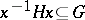. A special case of this is the following conjecture:

ZC2) Ifis a subgroup ofof augmentation one of ordersuch that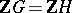, then there exists a unitwith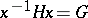. This last conjecture was earlier proved by K. Roggenkamp and L.R. Scott [a12] for nilpotent groups. Subsequently, they also gave a counterexample to ZC2) (unpublished), which appears in a modified form in [a5].

Clearly, ZC3) implies ZC1) and ZC2). Also, ZC2) implies that if two group ringsand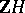are isomorphic, then the groupsandare isomorphic. This isomorphism problem was proposed in [a3]:(a1)

Of course, then, (a1) is true for nilpotent groups. Moreover, it was proved by A. Whitcomb [a18] that (a1) is true for metabelian groups. M. Hertweck [a2] has given a counterexample to (a1).

Conjecture ZC1) is open in general (as of 2000). Besides nilpotent groups, it is known to be true for certain split metacyclic groups [a10]: If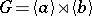is the semi-direct product of two cyclic groups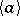andof relative prime orders, then ZC1) holds for.

This result has been strengthened to ZC3) [a15].

There are several useful and interesting extensions of the above conjectures. Suppose thatis a normal subgroup of indexin. Thencan be represented by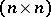-matrices over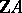. Any torsion unitofthat is mapped by the natural homomorphism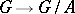to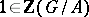gives rise to a torsion matrix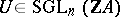. Here,denotes the subgroup of the general linear group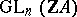consisting of the matricesthat are mapped by the augmentation homomorphism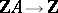, when applied to each entry, to the identity matrix. Thus, ZC1) translates to the question about diagonalization ofin: Is a torsion matrix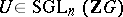, whereis a finite group, conjugate in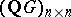to a matrix of the form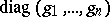,?

This was answered positively in [a16] for-groups (cf. also-group). See [a1] for an explicit example of a matrix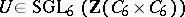that cannot be diagonalized but for which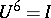. Such a matrixexists for a finite nilpotent groupand someif and only ifhas at least two non-cyclic Sylow-subgroups [a1] (cf. also Sylow subgroup).

However, it was proved in [a6] that ifandis finite Abelian, thenis conjugate into. This has been extended to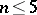in [a8], bridging the gap betweenand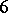.

The Zassenhaus conjectures and the isomorphism problem have also been studied for infinite groups. The statements remain the same and the groupis arbitrary. A counterexample to ZC1) was provided in [a9]. Conjecture ZC2) also does not hold for infinite groups, as shown by S.K. Sehgal and A.E. Zalesskii (see [a14], p. 279).

However, one can ask if any torsion unit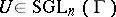can be stably diagonalized to,. This has been proved [a7] to be true for-elementswhenis nilpotent. The isomorphism problem also has a positive answer for finitely-generated nilpotent groups of class, cf. [a11]. In general for nilpotent groups the problem remains open (as of 2000).

How to Cite This Entry:
Zassenhaus conjecture. Encyclopedia of Mathematics. URL: http://encyclopediaofmath.org/index.php?title=Zassenhaus_conjecture&oldid=11419
This article was adapted from an original article by S.K. Sehgal (originator), which appeared in Encyclopedia of Mathematics - ISBN 1402006098. See original article